## Filters

Sort by :
Clear All
Q
Which of the species is more stable O or N? Nitrogen is more stable than Oxygen due to half-filled electrons in Nitrogen.

Both are active, but O is more active w.r.t. N due to its high electronegativity[ En(O) = 3.5 & En(N) = 3.0 ]. Hence, N is more stable w.r.t. O

In which case change in entropy is negative?

• Option 1)

Evapouration of water

• Option 2)

Expansion of a gas at constant temperature

• Option 3)

Sublimation of solid to gas

• Option 4)

Gibb's free energy (? G) -   - wherein Gibb's free energy enthalpy of reaction entropy temperature       degree of randomness decreases  entropy decreases and is negative.Option 1)Evapouration of waterOption 2)Expansion of a gas at constant temperatureOption 3)Sublimation of solid to gasOption 4)

Match the following:

(a) Pure Nitrogen                (i) Chlorine

(b) Haber process              (ii) Sulphuric Acid

(c) Contact process           (iii) Ammonia

(d) Deacon's process        (iv) Sodium azide or Barium Azide

Which of the following is the correct option?

(a)    (b)    (c)    (d)

• Option 1)

(i)    (ii)    (iii)    (iv)

• Option 2)

(ii)    (iv)    (i)    (iii)

• Option 3)

(iii)    (iv)    (ii)    (i)

• Option 4)

(iv)    (iii)    (ii)    (i)

Option 4 a) Pure Nitrogen  Sodium azide  or Barium azide  b) Haber process  Making ammonia                                        c) Contact process  producing sulphuric acid  d) Deacon's process  Pruduces  gas                                              Option 1)(i)    (ii)    (iii)    (iv)Option 2)(ii)    (iv)    (i)    (iii)Option 3)(iii)    (iv)    (ii)    (i)Option 4)(iv)    (iii)    (ii)    (i)

The mixture that forms maximum boiling azeotrope is:

• Option 1)

Water + Nitric Acid

• Option 2)

Ethanol + Water

• Option 3)

Acetone + Carbon disulphide

• Option 4)

Heptane + Octane

The Solutions that show large negative deviation from Raoult's law from maximum boiling azeotrope at a specific combination. Nitric acid and water is the example of this class of azeotrope.  68% Nitric acid + 32% by mass with a boiling point of 393.5 K.    Option 1)Water + Nitric AcidOption 2)Ethanol + WaterOption 3)Acetone + Carbon disulphideOption 4)Heptane + Octane

Among the following, the one that is not a greenhouse gas is:

• Option 1)

nitrous oxide

• Option 2)

methane

• Option 3)

ozone

• Option 4)

sulphur dioxide

N2O (Nitrous oxide) CH4 (Methane) O3 (Ozone) All the above are greenhouse gases but SO2  is not greenhouse gas.  Option 1) nitrous oxide Option 2) methane Option 3) ozone Option 4) sulphur dioxide

The correct structure of tribromooctaoxide is:

• Option 1)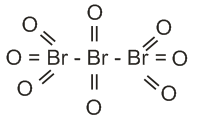• Option 2)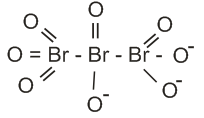• Option 3)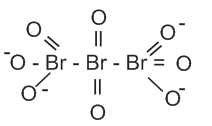• Option 4)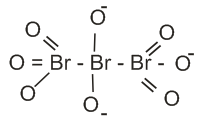option 1 ,because it is not an ion

The number of sigma  and pi bonds in pent-2-en-4-yne is:

• Option 1)

10  bonds and 3  bonds

• Option 2)

bonds and 5  bonds

• Option 3)

11  bonds and 2  bonds

• Option 4)

13  bonds and no  bonds

Option 1 Structure of pent-2-en-4-yne Here there are 10  bonds  and  bonds And 3  bonds   and Option 1)10  bonds and 3  bondsOption 2)8  bonds and 5  bondsOption 3)11  bonds and 2  bondsOption 4)13  bonds and no  bonds

An alkane "A" on reaction with O3 and Zn-H2O gives propane and ethanal in equimolar ratio. Addition of HCl to Alkane "A" gives "B" as the major product. The structure of the product "B" is

• Option 1)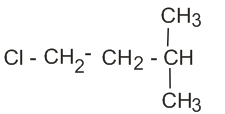• Option 2)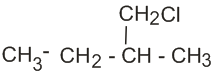• Option 3)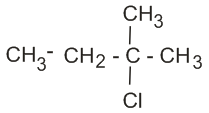• Option 4)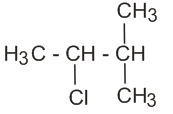This oxidation of alkene occurs at the double bond. Thus, propane & ethanol must combine to give the alkene             (Propanone)                                                                                                       Now this reacts with HCl to form B as follows:                                                                         ...

Match the Xenon compound in Column-I with its structure in Column-II and assign the correct code:

Column-I                        Column-II

(a) XeF4                            (i) pyramidal

(b) XeF6                            (ii) Square planar

(c) XeOF4                        (iii) distorted octahedral

(d) XeO3                          (iv) square pyramidal

Code:

(a)     (b)     (c)     (d)

• Option 1)

(i)     (ii)     (iii)     (iv)

• Option 2)

(ii)      (iii)     (iv)     (i)

• Option 3)

(ii)     (iii)     (i)     (iv)

• Option 4)

(iii)     (iv)     (i)     (ii)

XeF4  it is square planar. XeF6  it is distorted octahedral because of the lone pair present. XeOF4  Its is square pyramidal & its hybridization is sp3d2 XeO3  Its hybridization is sp3 & its shape is pyramidal.  (a) - (ii), (b) - (iii), (c) - (iv), (d) - (i) Option 2 is correct.Option 1)(i)     (ii)     (iii)     (iv)Option 2)(ii)      (iii)     (iv)     (i)Option 3)(ii)     (iii)     (i)   ...

Which mixture of the solution will lead to the formation of negatively charged colloidal  sol. ?

• Option 1)

50 mL of 1 M  + 50 mL of 1.5 M

• Option 2)

50 mL of 1 M  + 50 mL of 2 M

• Option 3)

50 mL of 2 M  + 50 mL of 1.5 M

• Option 4)

50 mL of 0.1 M  + 50 mL of 0.1 M

Option 2                     Negatively charged colloidal  need 2 mol of   Option 1)50 mL of 1 M  + 50 mL of 1.5 M Option 2)50 mL of 1 M  + 50 mL of 2 M Option 3)50 mL of 2 M  + 50 mL of 1.5 M Option 4)50 mL of 0.1 M  + 50 mL of 0.1 M

Which of the following is incorrect statement?

• Option 1)

PbF4 is covalent in nature

• Option 2)

SiCl4 is easily hydrolysed

• Option 3)

GeX4 (X = F, Cl, Br, I) is more stable than GeX2

• Option 4)

SnF4 is ionic in nature

(i) PbF4 is not covalent in nature. Because Pb atom is very & F atom is very small, so according to Fajan's rule, PbF4 is not covalent. (ii) SiCl4 is not a very stable compound because of steric hindrance thus can be hydrolysed very easily (iii) GeX4 is more stable than GeX2 as in GeX4 all the orbitals are fully filled.  (iv) SnF4 is ionic in nature. Because F atom is very small & Sn atom is...

What is the correct electronic configuration of the central atom in  based on crystal field theory?

• Option 1)

• Option 2)

• Option 3)

• Option 4)

Splitting occurs as follows for octahedral For Fe2+ (d6), electron distribution occurs as follows:  is the correct distribution. Option 2 is correct.  Option 1)Option 2)Option 3)Option 4)

The non-essential amino acid among the following is:

• Option 1)

valine

• Option 2)

leucine

• Option 3)

alanine

• Option 4)

lysine

2

For an ideal solution, the correct option is:

• Option 1)

at constant T and P

• Option 2)

at constant T and P

• Option 3)

at constant T and P

• Option 4)

at constant T and P

For an ideal solution, the Gibbs free energy is always negative, thus their mixing is always spontaneous. The volume change of the mixing of ideal solutions is zero. An ideal solution is one in which the enthalpy of the solution is zero  at constant T and P Option 3 is correct.Option 1) at constant T and POption 2) at constant T and POption 3)  at constant T and POption 4) at constant T and P

For the cell reaction

at 298K. The standard Gibbs energy  of the cell reaction is:

[Given that Faraday constant F = 96500 C mol-1]

• Option 1)

-46.32 kJ mol-1

• Option 2)

-23.16 kJ mol-1

• Option 3)

46.32 kJ mol-1

• Option 4)

23.16 kJ mol-1

We know that       Option 1 is correctOption 1)-46.32 kJ mol-1Option 2)-23.16 kJ mol-1Option 3)46.32 kJ mol-1Option 4)23.16 kJ mol-1

Enzymes that utilize ATP in phosphate transfer require an alkaline earth metal (M) as the cofactor M is:

• Option 1)

Be

• Option 2)

Mg

• Option 3)

Ca

• Option 4)

Sr

Option 2 Mg is the alkaline earth metal as the co-factor. (FACT)Option 1)BeOption 2)MgOption 3)CaOption 4)Sr

A compound is formed by cation C and anion A. The Anions form hexagonal close packed lattice and the cations occupy 75% of the octahedral void. The formula of the compound is

• Option 1)

• Option 2)

• Option 3)

• Option 4)

Option 3 Anions: No of ions per unit in HCP = 6 Cations: No of octahedral voids in HCP = 6                         So, 75% of 6 = 4.5     Option 1)Option 2)Option 3)Option 4)

A gas at 350K and 15 bar has molar volume 20 percent smaller than that for an ideal gas under the same conditions. The  correct  option about the gas and its compressibility factor (Z) is:

• Option 1)

Z>1 and attractive forces are dominant

• Option 2)

Z>1 and repulsive forces are dominant

• Option 3)

Z<1 and attractive forces are dominant

• Option 4)

Z<1 and repulsive forces are dominant

Option 3          Molar volume of gas is 20% smaller than that of the ideal gas. Hence Z < 1 and attractive forces are dominant. When Z < 1: Gas shows negative deviation and is more compressible than expected from ideal behaviour.  Z < 1 corresponds to attractive forces between molecules.Option 1)Z>1 and attractive forces are dominantOption 2)Z>1 and repulsive forces are dominantOption 3)Z<1...

• Option 1)

nylon-6,6

• Option 2)

nylon 2 - nylon 6

• Option 3)

nylon-6

• Option 4)

Buna-S

Option 2  Biodegradable polymer is Nylon-2-Nylon-6 monomers: Glycine, aminocaproic acid Option 1)nylon-6,6Option 2)nylon 2 - nylon 6Option 3)nylon-6Option 4)Buna-S

For the chemical reaction

the correct option is:

• Option 1)

• Option 2)

• Option 3)

• Option 4)

For a chemical reaction: The rate is always given as:  for the reaction: , Thus,  Option 3 is correct.Option 1)Option 2)Option 3)Option 4)
Exams
Articles
Questions